Health, Sports & Psychology

### Become an OU student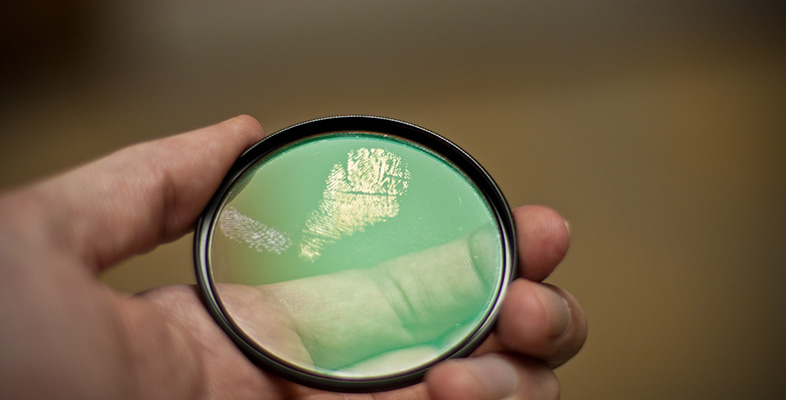Forensic science and fingerprints

Start this free course now. Just create an account and sign in. Enrol and complete the course for a free statement of participation or digital badge if available.

# Redox reactions

The second kind of chemical reaction that is used extensively in forensic science, and analytical science in general, is the redox reaction. Redox is a contraction of 'reduction and oxidation' and refers to reactions in which one molecule or ion is oxidised and the other reduced. One simple definition of oxidation is the addition of oxygen to a substance. There are lots of examples of an oxidation reaction where oxygen in the atmosphere reacts with something to form a new substance. For example, burning coal, which is carbon, causes it to combine with oxygen to form carbon dioxide (Equation 1) and burning methane gas (CH4) in air produces carbon dioxide and water (Equation 2). In our bodies, glucose (C6H12O6) reacts with the oxygen that we breathe in to produce the carbon dioxide that we breathe out and water (Equation 3).

The definition of oxidation in terms of addition of oxygen is not appropriate for all oxidation reactions, and oxidation is better understood in terms of the loss of electrons. Rusting of iron metal (Fe) is another familiar example of an oxidation reaction. Here, each iron atom loses three electrons to form an iron(III) (ferric) ion as shown in Equation 5, where the symbol eˉ is used to denote an electron and 3eˉ means three electrons. The symbol Fe3+ means that the iron nucleus has three more protons that it has electrons.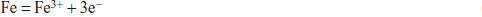Equation label: (Equation 5)

## Question 14

If an oxidation is loss of electrons, what do you think a reduction might be?

A reduction is where something gains electrons, such as that shown in Equation 6 where an aluminium ion is reduced to metallic aluminium. (This is the energy-intensive process used to make aluminium metal.)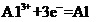Equation label: (Equation 6)

Another redox reaction, in which the copper atom is oxidised by the silver ion which is correspondingly reduced, is shown in Equation 7.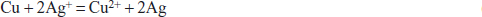Equation label: (Equation 7)

What this equation shows in chemical language is that if you dip, say, a copper coin into a solution containing silver ions then the copper atoms are oxidised by losing two electrons and the silver ions are simultaneously reduced with each one gaining an electron. The result is that the copper coin becomes silver plated. This is the principle behind Sheffield plating in which copper items such as candlesticks are coated in silver. A similar principle is used in a technique called 'physical developer', where oily or greasy fingerprints may be visualised by the deposition of metallic silver in a redox reaction (Equation 8).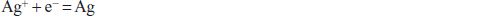Equation label: (Equation 8)

## Question 15

Is the reaction of the silver ion to form silver metal in Equation 8 an oxidation or a reduction?